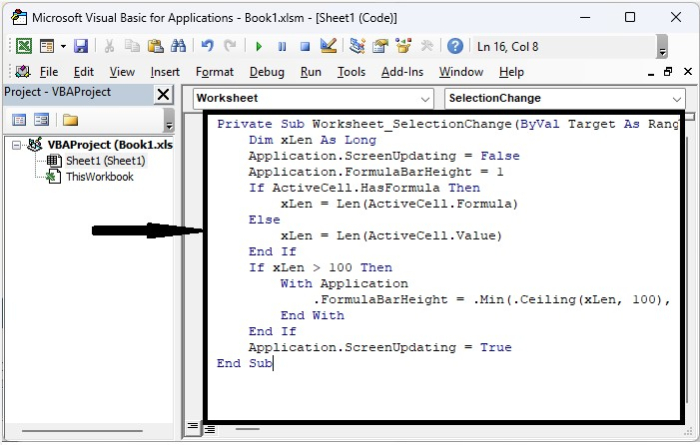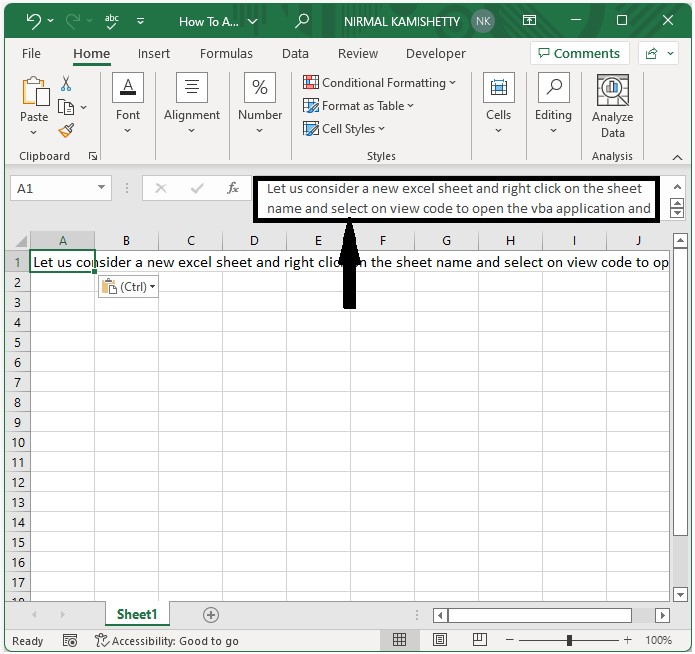# How to Automatically Expand the Formula Bar in Excel?

Entering the formula in a formula box is one of the most frequently used processes in Excel. You may have observed that when we enter a formula in the provided box, the formula will always be too small or too large for the formula we are using. This could create problems because sometimes we will not be able to see the whole formula that we are using, and sometimes the space in the formula box will be wasted, reducing our view of the data. We can solve the problem of formula visibility by automatically expanding the formula bar in Excel. This tutorial will help you understand how we can automatically expand the formula bar in Excel.

## Automatically Expand the Formula Bar in Excel

Here we will insert VBA code, and then we can see the formula box will be expanded. Let us see a straightforward process for how we can automatically expand the formula bar in Excel with the help of a VBA application.

Step 1

Consider creating a new Excel sheet and right-clicking on the sheet name to open the VBA application, then typing the programme into the textbox as shown in the image below.

### Example

Private Sub Worksheet_SelectionChange(ByVal Target As Range)
Dim xLen As Long
Application.ScreenUpdating = False
Application.FormulaBarHeight = 1
If ActiveCell.HasFormula Then
xLen = Len(ActiveCell.Formula)
Else
xLen = Len(ActiveCell.Value)
End If
If xLen > 100 Then
With Application
.FormulaBarHeight = .Min(.Ceiling(xLen, 100), 600) / 100
End With
End If
Application.ScreenUpdating = True
End SubStep 2

Save the sheet as a macro-enabled template and exit the vba application by pressing "Alt + Q". From now on, if something does not fit in the sheet, the bar will expand automatically, as shown in the below image. The size of the box will always depend on the amount of text we have entered in the formula box.## Conclusion

In this tutorial, we used a simple example to demonstrate how we can automatically expand the formula bar in Excel.